Home MATHEMATICS TOPIC 6: GEOMETRY | MATHEMATICS FORM 1

# TOPIC 6: GEOMETRY | MATHEMATICS FORM 1

4501
150## TOPIC 6: GEOMETRY

Points and Lines

The Concept of a Point

Explain the concept of a point

A​​ point​​ – is a smallest geometric figure which gives a position of object in a plane

A line segment – is a straight line joining two points in a plane

The Concept of a Point to Draw a Line

Extend the concept of a point to draw a line

A line segment – is a straight line joining two points in a plane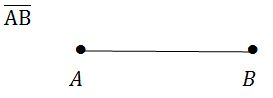A line passing through two points e.g A and B and extends without end​​ (i.e infinitely)​​ in both directions is denoted by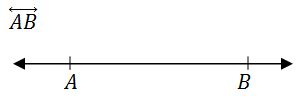The Difference Between a Line, a Line Segment and a Ray

Distinguish between a line, a line segment and a ray

A ray – is a line starting from a point, say A and pass through a point, say B and extends without end in one direction.It is denoted by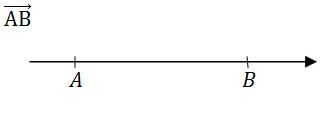#### Angles and Lines

Angles

Draw angles

An angle – is a measure of an amount of turn. For instance, a complete turn has an angle of 360º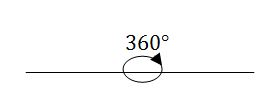Measuring Angles of Different Size Using a Protractor

Measure angles of different size using a protractor

There are several types of angles including:- acute, right, complementary, obtuse, supplementary and reflex angle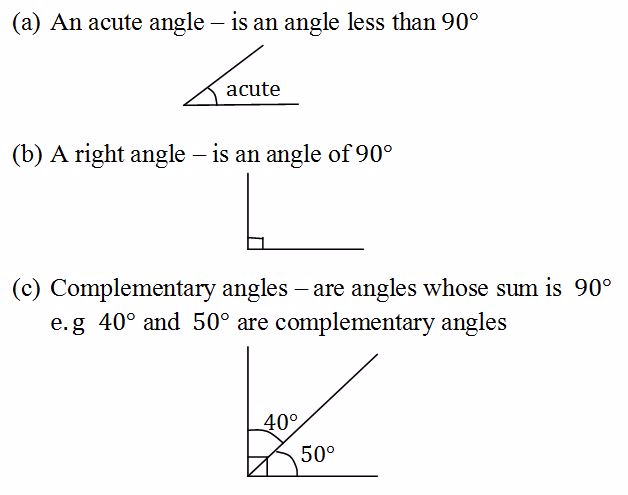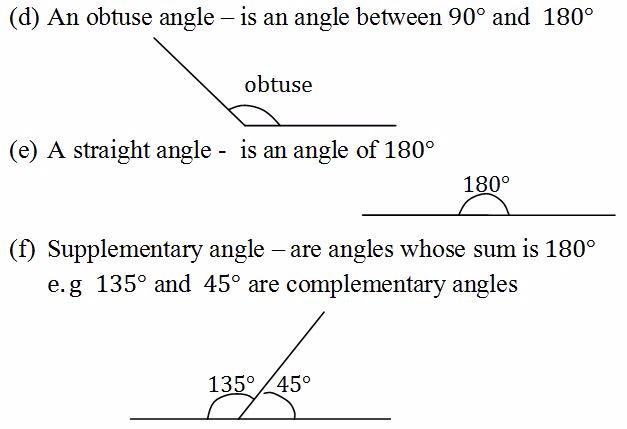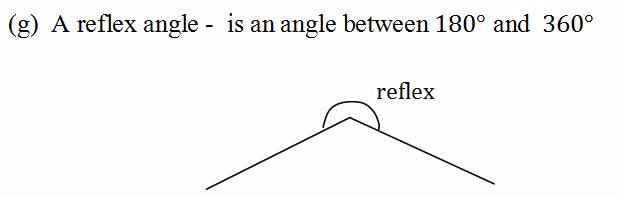Example 1

Two angles are supplementary. One angle is three times the other. What are the angles?

Two angles are complementary. One angle is 40º greater than the other. What are the angles?

Solution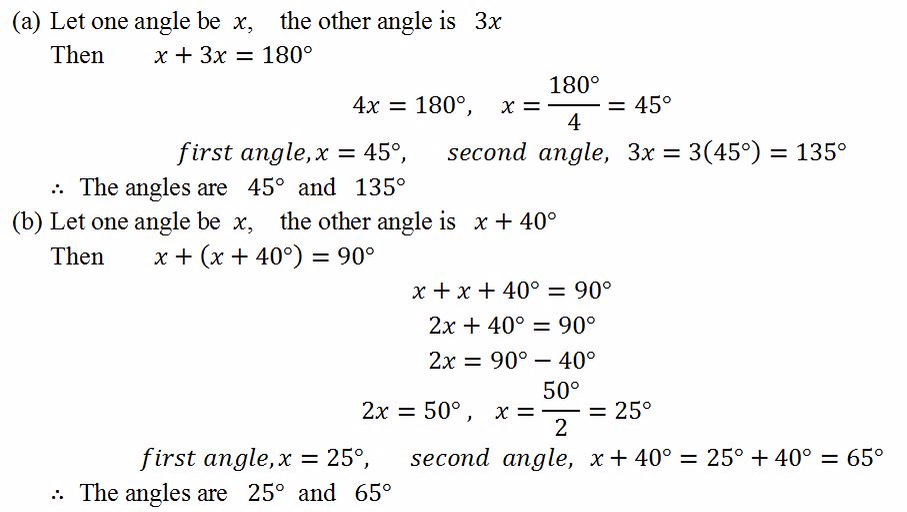#### Drawing Angles Using a Protractor

Draw angles using a protractor

The angles formed by crossing lines includes vertically opposite angles, alternate angle and corresponding angles

Vertically opposite angles

The angles on the opposite sides of the crossing lines are equal